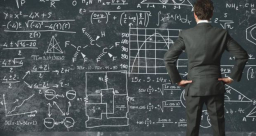# Two numbers 11

The sum of two rational numbers is (-2). If one of them is 3/5, find the other.

y =  -2.6

### Step-by-step explanation:Did you find an error or inaccuracy? Feel free to write us. Thank you!Tips to related online calculators
Need help to calculate sum, simplify or multiply fractions? Try our fraction calculator.
Do you have a linear equation or system of equations and looking for its solution? Or do you have a quadratic equation?

## Related math problems and questions:

• Sum of 18Sum of two fractions is 4 3/7. If one of the fractions is 2 1/5 find the other one .3 3/4 + 2 3/5 + 5 1/2 Show your solution.
• Fractions and mixed numerals(a) Convert the following mixed numbers to improper fractions. i. 3 5/8 ii. 7 7/6 (b) Convert the following improper fraction to mixed number. i. 13/4 ii. 78/5 (c) Simplify these fractions to their lowest terms. i. 36/42 ii. 27/45 2. evaluate following ex
• Two numbers and its productThe product of two numbers are 2/3. If on of them is 1/10, what is the other?
• If-then equationIf 1/2 + 2/5s = s - 3/4 what is the value of s?
• The sum 12The sum of 3 mixed numbers is 20 13/15. two of the numbers are 6 1/3 and 7 5/6. what is the third number?
• The product 2The product of two functions is 10. If one of them is 2 1/3, find the other one.
• Find unknown 2Find unknown denominator: 2/3 -5/? = 1/4
• Quotient and productIf the quotient of [8/5 divided by 8/10] is added to the product of [8/14 x 7/12 x 3/8], what is the sum?
• Sum of fractionsWhat is the sum of 2/3+3/5?
• Sum of 20Sum of the two numbers is 15 1/6. If one of the numbers is 2 2/3, find the other number.
• What is 9What is the value of x in the proportion 2 and one-fourth over x = 1 and one-half over 3 and three-fifths? 2 and two-fifths 5 and two-fifths 8 and 1 over 10 12 and 3 over 20
• Fraction of a NumberIf 1/2 of 1/3 of 1/4 of 1/5 of a number is 2.5. what is the number?
• Simplify 3Simplify mixed numerals expression: 8 1/4- 3 2/5 - (2 1/3 - 1/4) Show your solution.
• Complicated sum minus productWhat must be subtracted from the sum of 3/8 and 5/16 to get difference equal to the product of 5/8 and 3/16?
• 5 2/55 2/5 hours a week  mathematics,  3 3/4 hours a week   Natural sciences, 4 3/8 hours a week  Technology . how many hours does  he spend on social sciences if he spend 17 1/2 hours a week for the four subject?
• Product of two fractionsProduct of two fractions is 9 3/5 . If one of the fraction is 9 3/7. Find the other fraction.